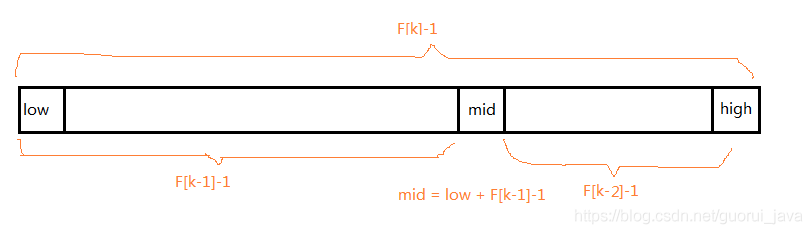# 【数据结构与算法 12】二分查找，java 大数据分析技术栈temp++;

}

return resIndexlist;

}

}

### 2、mid 变量的计算

mid=(left+right)/2；

mid=left + (right - left)*(findVal?- arr[left])/(arr[right]-arr[left])；

### 3、代码实例

public static int insertValueSearch(int[] arr, int left, int right, int findVal){

if(left>right || findVal<arr[left] || findVal>arr[right]){

return -1;

}

int mid = left + (right - left)*(findVal - arr[left])/(arr[right]-arr[left]);

int midVal = arr[mid];

if(findVal>midVal){

return insertValueSearch(arr,mid + 1,right,findVal);

}else if(findVal>midVal){

return insertValueSearch(arr,left,mid - 1,findVal);

}else {

return mid;

}

}

### 4、插值查找算法和二分查找算法对比

（1）对于数据量较大，数值分布比较均匀的数组来说，使用插值查找算法，速度更快一些；

（2）但是对于数值分布不均匀的数组来说，建议使用二分查找算法；

### 1、基本思想

{1,1,2,3,5,8,13,21,55}，发现规律了吗，前一个数值除以后一个数值，无限接近 0.618，这个数列就称为斐波那契数列。

### 2、mid 值的计算

mid=left + fibonacciArr[f-1] -1;

fibonacciArr：表示斐波那契数组；

f：表示斐波那契数列的第 f 个元素；

fibonacciArr[f] =?fibonacciArr[f-1] +?fibonacciArr[f-2];### 3、代码实现

//因为后面我们 mid=low+F(k-1)-1，需要使用到斐波那契数列，因此我们需要先获取到一个斐波那契数列

//非递归方法得到一个斐波那契数列

public static int[] fibonacci(){

int[] fibonacci = new int;

fibonacci = 1;

fibonacci = 1;

for (int i = 2; i < fibonacci.length - 1; i++) {

fibonacci[i] = fibonacci[i-1] + fibonacci[i-2];

}

return fibonacci;

}

//编写斐波那契查找算法

//使用非递归的方式编写算法

public static int fibonacciSearch(int[] arr,int findVal){

int left = 0;

int right = arr.length - 1;

int f = 0;//表示斐波那契分割数值的下标

int mid = 0;

int[] fibonacci = fibonacci();

//获取到斐波那契分割数值的下标

while (right > fibonacci[f] - 1){

f++;

}

//因为 f[f] 值 可能大于 arr 的 长度，因此我们需要使用 Arrays 类，构造一个新的数组，并指向 temp[]

//不足的部分会使用 0 填充

int[] temp = Arrays.copyOf(arr,fibonacci[f]);

//实际上需求使用 arr 数组最后的数填充 temp

//举例:

//temp = {1,8, 10, 89, 1000, 1234, 0, 0} => {1,8, 10, 89, 1000, 1234, 1234, 1234,}

for (int i = right + 1; i < temp.length; i++) {

temp[i] = arr[right];

}

// 使用 while 来循环处理，找到我们的数 findVal

whi

【一线大厂Java面试题解析+核心总结学习笔记+最新架构讲解视频+实战项目源码讲义】浏览器打开：qq.cn.hn/FTf 免费领取

le (left <= right) {

//fibonacci 寻找 mid 固定写法

mid = left + fibonacci[f - 1] - 1;

if (findVal < temp[mid]) { //我们应该继续向数组的前面查找(左边)

right = mid - 1;

//为甚是 f--

//说明

//1. 全部元素 = 前面的元素 + 后边元素

//2. fibonacci[f] = fibonacci[f-1] + fibonacci[f-2]

//因为 前面有 fibonacci[f-1]个元素,所以可以继续拆分 fibonacci[f-1] = fibonacci[f-2] + fibonacci[f-3]

//即 在 fibonacci[f-1] 的前面继续查找 f--

//即下次循环 mid = fibonacci[f-1-1]-1

f--;

} else if (findVal > temp[mid]) { // 我们应该继续向数组的后面查找(右边)

left = mid + 1;## 评论